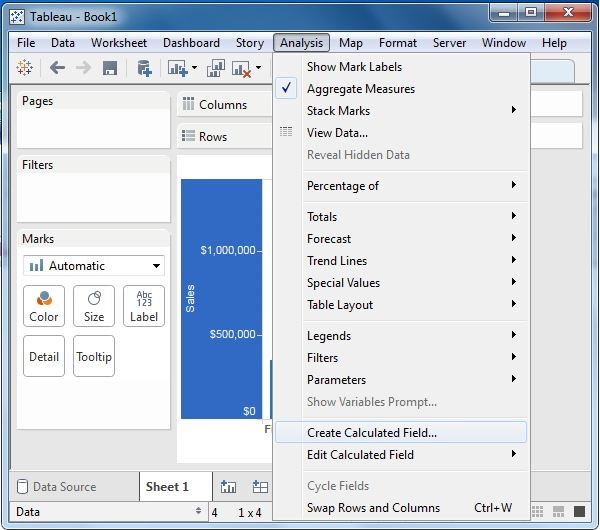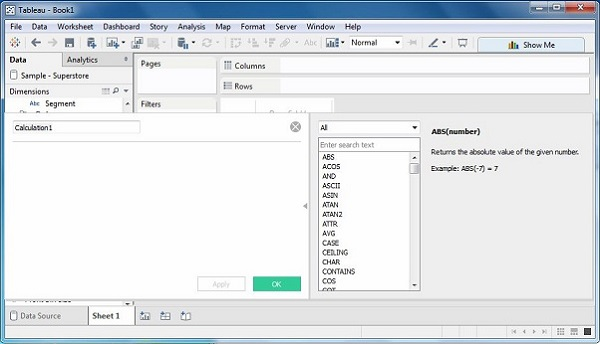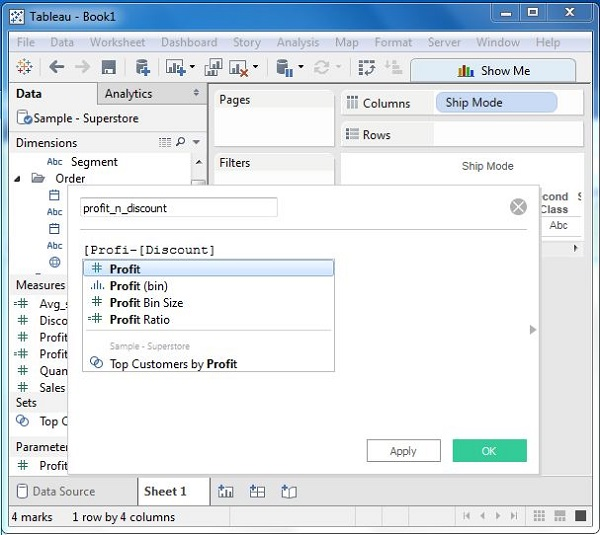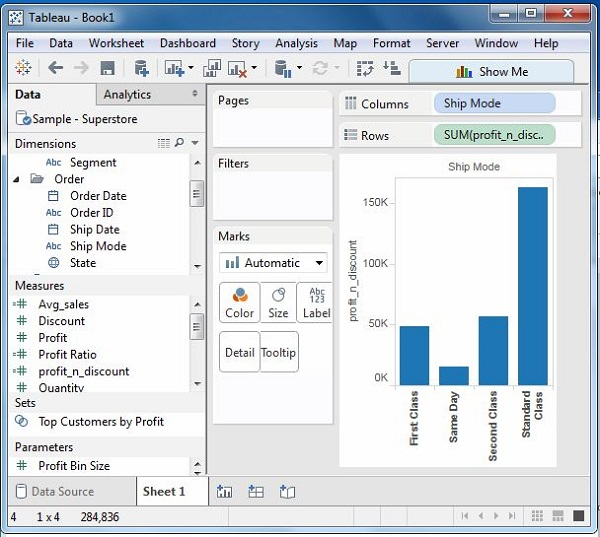# Tableau - Numeric Calculations

Numeric calculations in Tableau are done using a wide range of inbuilt functions available in the formula editor.

In this chapter, we will see how to apply calculations to the fields. The calculations can be as simple as subtracting the values of two fields or applying an aggregate function to a single field.

Following are the steps to create a calculation field and use numeric functions in it.

## Create Calculated Field

While connected to Sample-superstore, go to the Analysis menu and click ‘Create Calculated Field’, as shown in the following screenshot.## Calculation Editor

The above step opens a calculation editor which lists all the functions that is available in Tableau. You can change the dropdown value and see only the functions related to numbers.## Create a Formula

To study the difference between profit and discount for different shipping mode of the products, create a formula subtracting the discount from the profit as shown in the following screenshot. Also, name this field as profit_n_discount.## Using the Calculated Field

The above calculated field can be used in the view by dragging it to the Rows shelf as shown in the following screenshot. It produces a bar chart showing the difference between profit and discount for different shipping modes.## Applying Aggregate Calculations

In a similar manner as above, you can create a calculated field using aggregate function. Here, create AVG(sales) values for different ship mode. Write the formula in the calculation editor as shown in the following screenshot.On clicking OK and dragging the Avg_Sales field to the Rows shelf, you will get the following view.